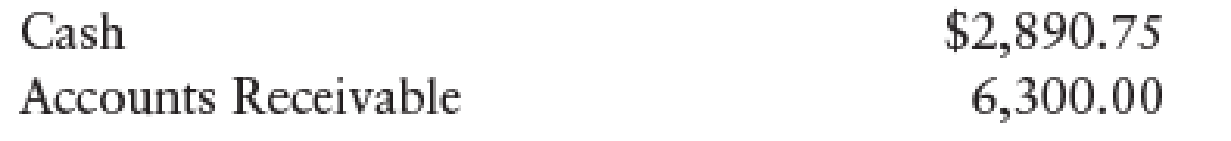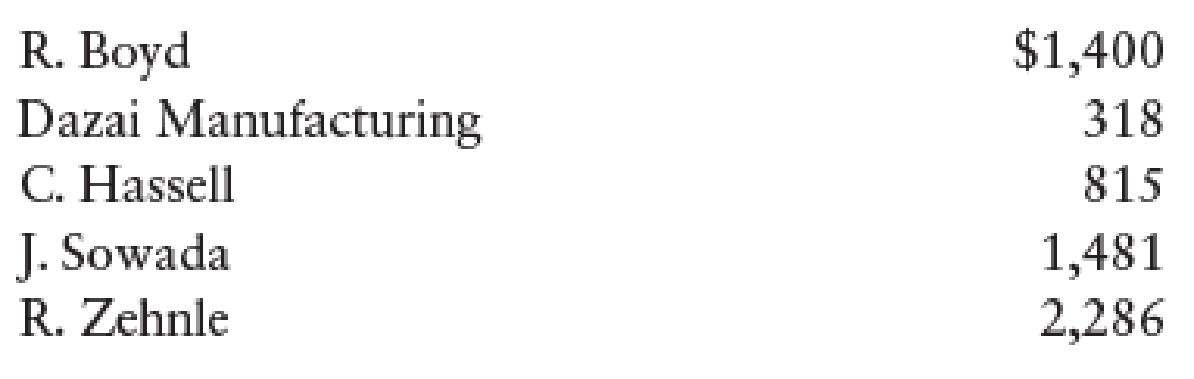Chapter 12, Problem 7SPB

Chapter
Section
Textbook Problem

CASH RECEIPTS JOURNAL Color Florists, a retail business, had the following cash receipts during January 20--. The sales tax is 5%.Jan. 1    Received payment on account from Ray Boyd, $880.3 Received payment on account from Clint Hassell,$271.5    Made cash sales for the week, $2,800, plus tax. Bank credit card sales for the week,$1,200, plus tax. Bank credit card fee is 3%.8    Received payment on account from Jan Sowada, $912.11 Ray Boyd returned merchandise for a credit,$40, plus tax.12    Made cash sales for the week, $3,100, plus tax. Bank credit card sales for the week,$1,900, plus tax. Bank credit card fee is 3%.15    Received payment on account from Robert Zehnle, $1,100.18 Robert Zehnle returned merchandise for a credit,$31, plus tax.19    Made cash sales for the week, $2,230, plus tax.25 Received payment on account from Dazai Manufacturing,$318.Beginning general ledger account balances were as follows:Beginning customer account balances were as follows:Required 1. Record the transactions in the cash receipts journal. Total and verify the column totals and rule the columns. Use the general journal to record sales returns and allowances. 2. Post from the journals to the general ledger and accounts receivable ledger accounts. Use account numbers as shown in the chapter.

1.

To determine

Prepare the given transactions in the cash receipts journal and verify the total column and rule the column and use the general journal to record the sales returns and allowances.

Explanation

Cash Receipts Journal:  It is a special book where only cash receipts transactions that are received from customers, merchandise sales and service made in cash and collection of accounts receivable are recorded.

The following are the some examples of transactions that would be recorded in the Other Accounts credit column of the cash receipts journal:

• • Cash received as interest on notes payable
• • Interest revenue received from debtors
• • Cash receipts from bank loans
• • Cash receipts for capital investments

Prepare the given transactions in the cash receipts journal and verify the total column and rule the column and use the general journal to record the sales returns and allowances:

Table (1)

Verification of total debit and credit column:

Total Debit     = Total Credit($15,174.85+$97.65)=($3,481.00+$11,230.00+$561.50)=$15,272.50=$15,272.5 Working note 1: Calculate the amount of cash on dated 5th January: Cash(debit)on dated 5thJanuary}=(Cash sales + 5% on sales tax)=[$2,800+(5%×$2,800)]=[$2,800+$140]=$2,940

Working note 2:

Calculate the amount of bank credit card expense on dated 5th January:

Bank credit card expense on dated 5thJanuary}=[(Sales + 5% on sales tax)×Credit card fee %]=[$1,200+(5%×$1,200)]×3%=[$1,200+$60]×3%=$37.80 Working note 3: Calculate the amount of cash on dated 5th January: Cash(debit)on dated 5thJanuary}=[(Cash sales + 5% on sales tax)Bank credit card expense]=[$1,200+(5%×$1,200)$37.80]=[$1,200+$60$37.80]=$1,222

Working note 4:

Calculate the amount of cash on dated 12th January:

Cash(debit)on dated 12thJanuary}=(Cash sales + 5% on sales tax)=[$3,100+(5%×$3,100)]=[$3,100+$155]=$3,255 Working note 5: Calculate the amount of bank credit card expense on dated 12th January: Bank credit card expense on dated 12thJanuary}=[(Sales + 5% on sales tax)×Credit card fee %]=[$1,900+(5%×$1,900)]×3%=[$1,900+$95]×3%=$59

2.

To determine

Post the prepared journal to the general ledger, and to the accounts receivable ledger.

Still sussing out bartleby?

Check out a sample textbook solution.

See a sample solution

The Solution to Your Study Problems

Bartleby provides explanations to thousands of textbook problems written by our experts, many with advanced degrees!

Get Started

Find more solutions based on key concepts